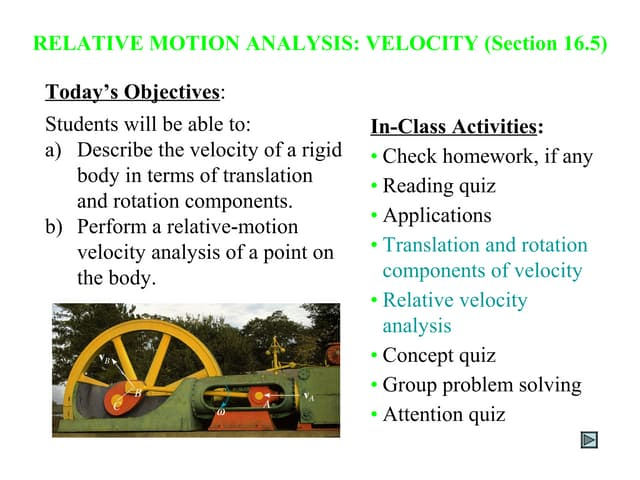PublicitéProchain SlideShareLecture16 5
Chargement dans ... 3
1 sur 2
Publicité

### Ball B- moving in the positive direction of an x axis at speed v- coll.docx

1. Ball B, moving in the positive direction of an x axis at speed v, collides with stationary ball A at the origin. A and B have different masses. After the collision, B moves in the positive direction of the y axis at speed v/4 (a) In what direction does A move? 11.3 xcounterclockwise from the +x axis (b) Show that the speed of A cannot be determined from the given information. (Select all that apply.) O The initial speed v is unknown The final direction of A is unknown. O The final position of A is unknown. The mass of A relative to the mass of B is unknown. O The final position of B is unknown Additional Materials Section 9.8 Solution Solution- As we know that momentum is conserved. Initially no momentum along the y-axis, Therefore A has the opposite y momentum from B. m_A v_y_A = m_B v/3 v_y_A = (m_B / 3m_A) v There WAS momentum along the y-axis, and B takes none of that, so A has all of its original xÂ Â momentum . m_A v_x_A = m_A v v_x_A = v The angle is related to the x and y components of velocity: tan ? = v_y_A / v_x_A ? = arctan (m_B / 3m_A) We can find direction as ?=arctan(1/4) =14.04 b) Here negative sign says that angle is clockwise below the x-axis from the above equation it is clear that to find the velocity of A, we need the mass of A and B Hence the given information is not sufficient.
Publicité